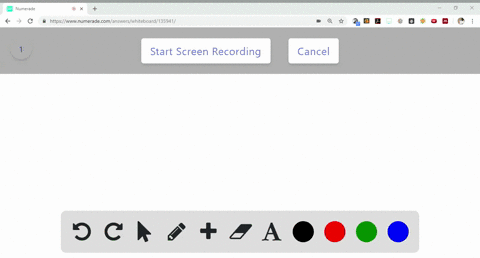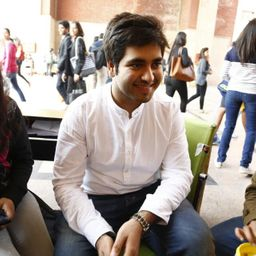Sign up for our free STEM online summer camps starting June 1st!View Summer Courses### Balance the following equations: $$\begin{arra… 12:00University of New Mexico Need more help? Fill out this quick form to get professional live tutoring. Get live tutoring Problem 11 Balance the following equations:$$
\begin{array}{l}{\text { (a) } \mathrm{CO}(g)+\mathrm{O}_{2}(g) \longrightarrow \mathrm{CO}_{2}(g)} \\ {\text { (b) } \mathrm{N}_{2} \mathrm{O}_{5}(g)+\mathrm{H}_{2} \mathrm{O}(l) \longrightarrow \mathrm{HNO}_{3}(a q)} \\ {\text { (c) } \mathrm{CH}_{4}(g)+\mathrm{Cl}_{2}(g) \longrightarrow \mathrm{CCl}_{4}(l)+\mathrm{HCl}(g)} \\ {\text { (d) } \mathrm{Zn}(\mathrm{OH})_{2}(s)+\mathrm{HNO}_{3}(a q) \longrightarrow \mathrm{Zn}\left(\mathrm{NO}_{3}\right)_{2}(a q)+\mathrm{H}_{2} \mathrm{O}(l)}\end{array}


a) See explanation for solution.
b) See explanation for solution.
c) See explanation for solution.
d) See Explanation.

## Discussion

You must be signed in to discuss.

## Video Transcript

for auction, eh? In this equation, oxygen atoms are known balance. There are three oxygen atoms on the react in sight by only two oxygen atoms are on the broader side multiplied the C o on the reactant side. Unconverted on convert, See or two on the product side by two. So the reaction will be to see oh, plus or two. We'll give this to see you too or option B in this equation. Oxygen, hydrogen and nitrogen on. On balance, begin with the oxygen atom. There are six oxygen atoms on the reactant side, but only three oxygen atoms are on the product side. Multiply at, you know, three on the reactant side by two. Then the oxygen atoms are balanced, giving us equation and two 05 Blessed itch, too. Oh, giving us to Etch and No. Three for Option C in this secretion. Hydrogen and chlorine atoms on. On balance, there are four hydrogen atoms on the reactant side, but only one hydrogen atom on the product side multiplied. The ex steel on the product side with four giving us equation sietch four plus seal, too, giving us see steal four plus four edged steel Now There are two chlorine atoms on the reactor inside, and eight chlorine atoms on the product. Multiply the chlorine molecule on the reactant side by four atoms to balance de chlorine atom, giving us the second part off equation ch four plus full seal, too. See seal four plus four Xia for last option Auction number D here in equation. Oxygen nitrogen on hydrogen are not balanced. Begin with the nitrogen atoms giving us the equation. Zinc, which two nest toe edge and No. Three leading us to is there did and no three balls, two plus edge to O. Now Multiply water molecule on the product side by two to balance out the hydrogen atom, giving us the second part of the equation. Zaidan, which two less two at general three leading to Zidan and 32 blessed toe edge, too. Oh,